# Mie potential

Jump to: navigation, search

The Mie potential was proposed by Gustav Mie in 1903 . It is given by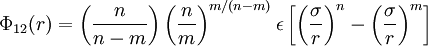$\Phi_{12}(r) = \left( \frac{n}{n-m}\right) \left( \frac{n}{m}\right)^{m/(n-m)} \epsilon \left[ \left(\frac{\sigma}{r} \right)^{n}- \left( \frac{\sigma}{r}\right)^m \right]$

where:

•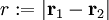$r := |\mathbf{r}_1 - \mathbf{r}_2|$
•$\Phi_{12}(r)$ is the intermolecular pair potential between two particles at a distance r;
•$\sigma$ is the value of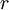$r$ at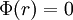$\Phi(r)=0$ ;
•$\epsilon$ : well depth (energy)

Note that when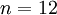$n=12$ and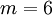$m=6$ this becomes the Lennard-Jones model.

The location of the potential minimum is given by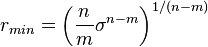$r_{min} = \left( \frac{n}{m} \sigma^{n-m} \right) ^ {1/(n-m)}$

## Second virial coefficient

The second virial coefficient    and the Vliegenthart–Lekkerkerker relation .